Learning Library

New Year, New Start: 15 Math Worksheets That Sharpen Skills

Start the new year off by getting back to basics. Here's a collection of review worksheets that get kids re-acquainted with simple addition and subtraction.

Click on a worksheet in the set below to see more info or download the PDF.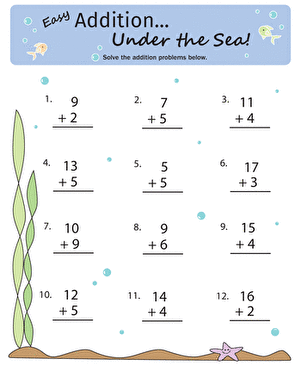Addition Under the Sea

Dive into addition with the single digits in this worksheet, featuring an ocean floor background.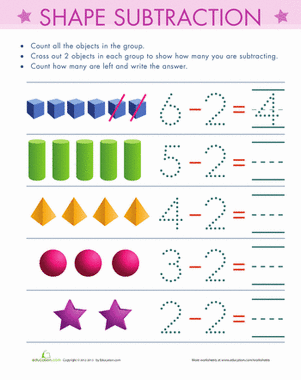Count and Subtract

Ready to introduce your child to subtraction, but you're not sure if he's ready? This worksheet is a simple and easy introduction.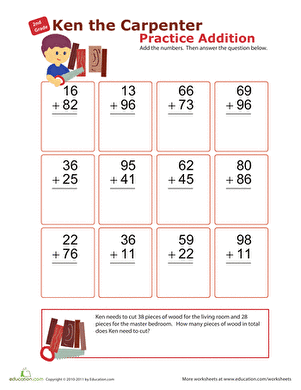Practice Addition with Ken the Carpenter!

Ask your second grader to help out Ken the Carpenter, and his double-digit addition skills will get a boost, too!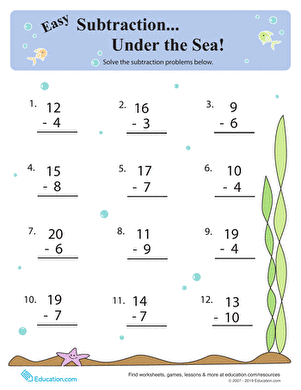Subtraction Under the Sea

Keep your first grade math whiz sharp with this ocean-themed worksheet practicing single-digit subtraction.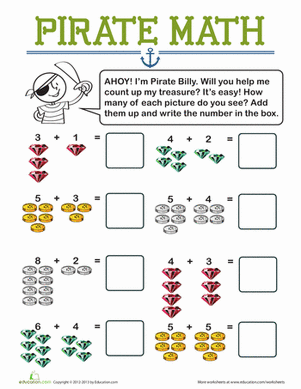Pirate Math

Ahoy! Pirate Billy needs help counting his treasures. Help your kid practice adding single-digit numbers and writing the sums on this treasure-themed worksheet.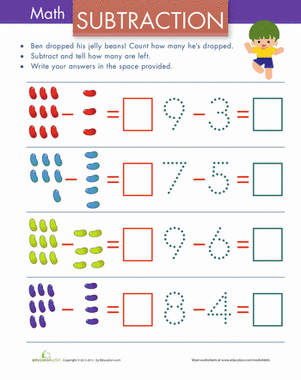Subtraction with Pictures: Jelly Beans

How many jelly beans were dropped? Help your little math whiz count her way to the difference of each subtraction equation.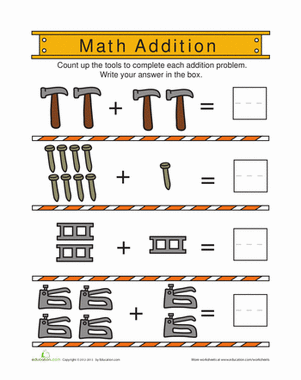Little builders, get ready for some construction zone math! Your child will use his counting skills to solve these addition equations.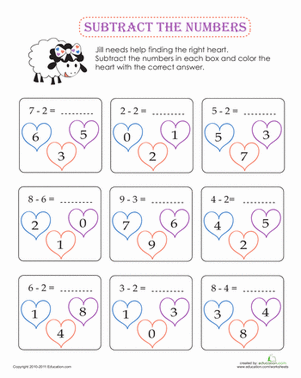Subtraction Coloring

Help Jill the Sheep track down the right heart by completing the subtraction problems in each box.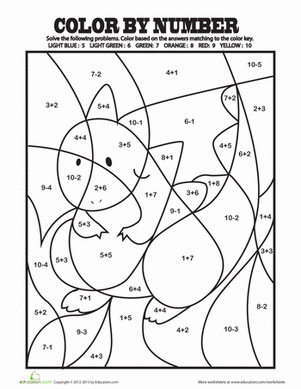Color by Addition Facts

Challenge your child with some basic addition and subtraction problems. Then she can use the answers to color by number her own work of art!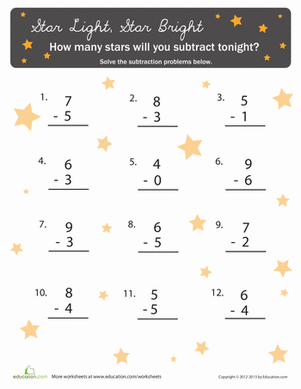Subtracting with Stars

Need to add some sparkle to your child's subtraction practice? Let the stars guide your math whiz as he tackles 12 problems amidst the stars.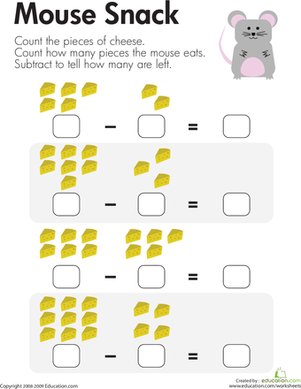Counting Up Subtraction

For each problem on this first grade math worksheet, kids subtract single-digit numbers to see how many cheese pieces are left after the mouse eats its snack.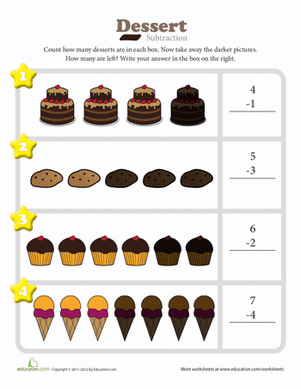Dessert Subtraction

Count the number of desserts in each box then take away the ones that are darker. How many are left?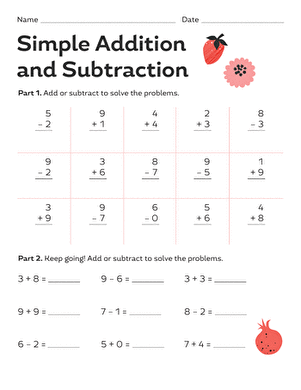Simple Addition and Subtraction

Lend your first grader a hand with beginning math. She'll practice both addition and subtraction with both vertical and horizontal equations.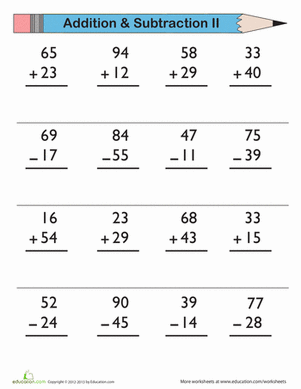Keep those math skills sharp with a little practice. All you have to do is add and subtract these double-digit numbers.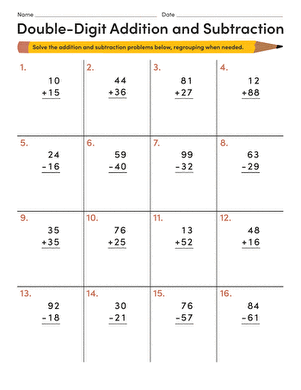Double-Digit Addition and Subtraction

Practice makes perfect! Use this worksheet for a quick practice session in double-digit addition and subtraction for your 1st grader.

Want to download this whole set as a single PDF?

Create new collection

0

New Collection>

0 items

What could we do to improve Education.com?

Please note: Use the Contact Us link at the bottom of our website for account-specific questions or issues.

What would make you love Education.com?